# Dynamic Programming: Ninja’s Training (DP 7)

Introduction To 2D Dynamic Programming / Ninja Training

In this article, we will understand the concept of 2D dynamic programming. We will use the problem ‘Ninja Training’ to understand this concept.

Pre-req: Dynamic Programming Introduction

Problem Link: Ninja Training

A Ninja has an ‘N’ Day training schedule. He has to perform one of these three activities (Running, Fighting Practice, or Learning New Moves) each day. There are merit points associated with performing an activity each day. The same activity can’t be performed on two consecutive days. We need to find the maximum merit points the ninja can attain in N Days.

We are given a 2D Array POINTS of size ‘N*3’ which tells us the merit point of specific activity on that particular day. Our task is to calculate the maximum number of merit points that the ninja can earn.

```Example: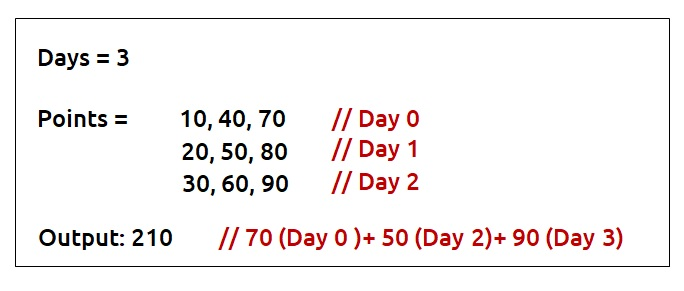```

Disclaimer: Don’t jump directly to the solution, try it out yourself first.

Pre-req: Recursion

### Solution :

Why a Greedy Solution doesn’t work?

The first approach that comes to our mind is the greedy approach. We will see with an example how a greedy solution doesn’t give the correct solution.

Consider this example: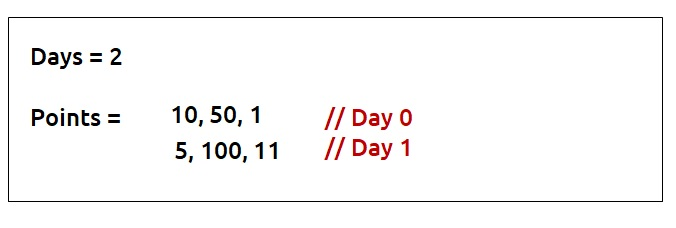We want to know the maximum amount of merit points. For the greedy approach, we will consider the maximum point activity each day, respecting the condition that activity can’t be performed on consecutive days.

• On Day 0, we will consider the activity with maximum points i.e 50.
• On Day 1, the maximum point activity is 100 but we can’t perform the same activity in two consecutive days. Therefore we will take the next maximum point activity of 11 points.
• Total Merit points by Greedy Solution : 50+11 = 61

As this is a small example we can clearly see that we have a better approach, to consider activity with 10 points on day0 and 100 points on day1. It gives us the total merit points as 110 which is better than the greedy solution.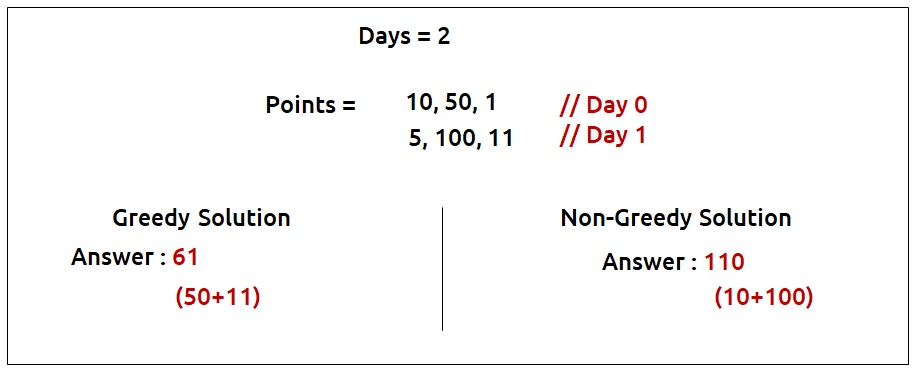So we see that the greedy solution restricts us from choices and we can lose activity with better points on the next day in the greedy solution. Therefore, it is better to try out all the possible choices as our next solution. We will use recursion to generate all the possible choices.

Steps to form the recursive solution:

We will first form the recursive solution by the three points mentioned in Dynamic Programming Introduction

Step 1: Express the problem in terms of indexes.

Now given an example, what is the one clear parameter which breaks the problem in different steps?
It is the number of days. Clearly, we have n days (from 0 to n-1), so one changing parameter which can be expressed in terms of indexes is ‘day’.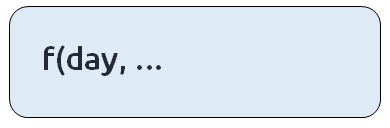Every day we have the option of three activities(say 0,1,2) to be performed. Suppose that we are at a day_i ( shown in the fig) in the example given below. What is one more parameter along with the day that we must know to try out the correct choices at day_i?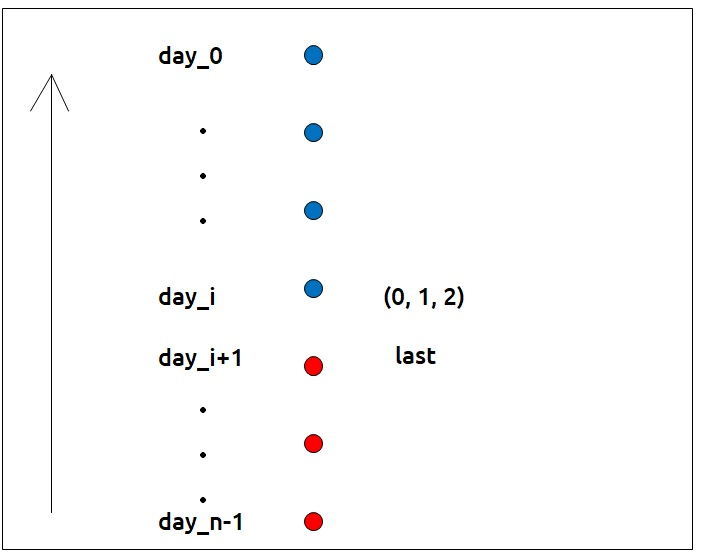It is the ‘last’ choice that we tried out on day_i+1 ( i+1 in case of top-down recursion). Unless we know the last choice we made, how can we decide whether a choice we are making is correct or not?
Now there are three options each day(say 0,1,2) which becomes the ‘last’ of the next day. If we are just starting from the day_n-1, then for the day_n-1 we can try all three options. We can say ‘last’ for day_n-1 is 3.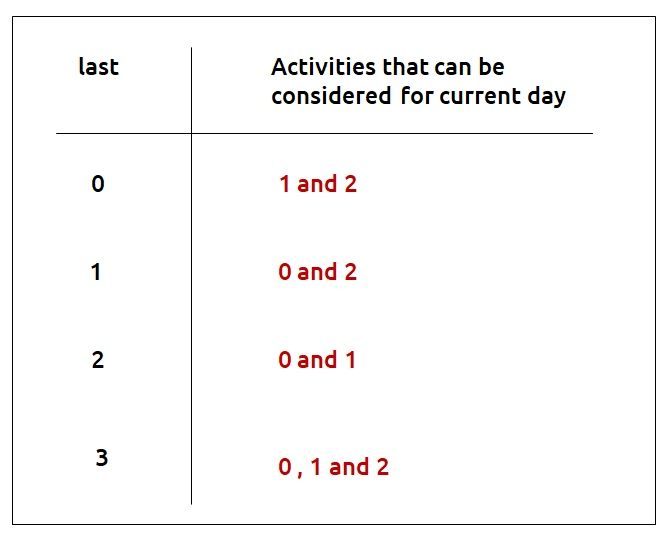Therefore our function will take two parameters – day and last.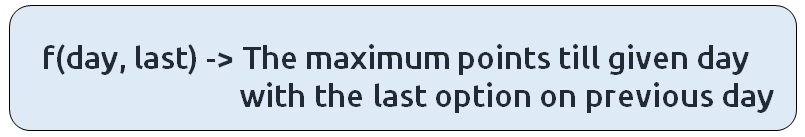Step 2: Try out all possible choices at a given index.

We are writing a top-down recursive function. We start from day_n-1 to day_0. Therefore whenever we call the recursive function for the next day we call it for f(day-1, //second parameter).
Now let’s discuss the second parameter. The choices we have for a current day depend on the ‘last’ variable. If we are at our base case (day=0), we will have the following choices.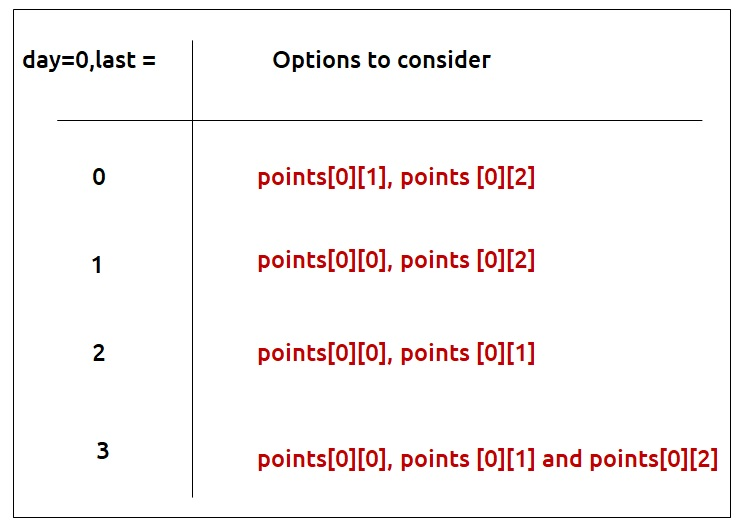Other than the base case, whenever we perform an activity ‘i’ its merit points will be given by points[day][i] and to get merit points of the remaining days we will let recursion do its job by passing f(d-1, i). We are passing the second parameter as i because the current day’s activity will become the next day’s last.

Step 3:  Take the maximum of all choices

As the problem statement wants us to find the maximum merit points, we will take the maximum of all choices.

The final pseudocode after steps 1, 2, and 3: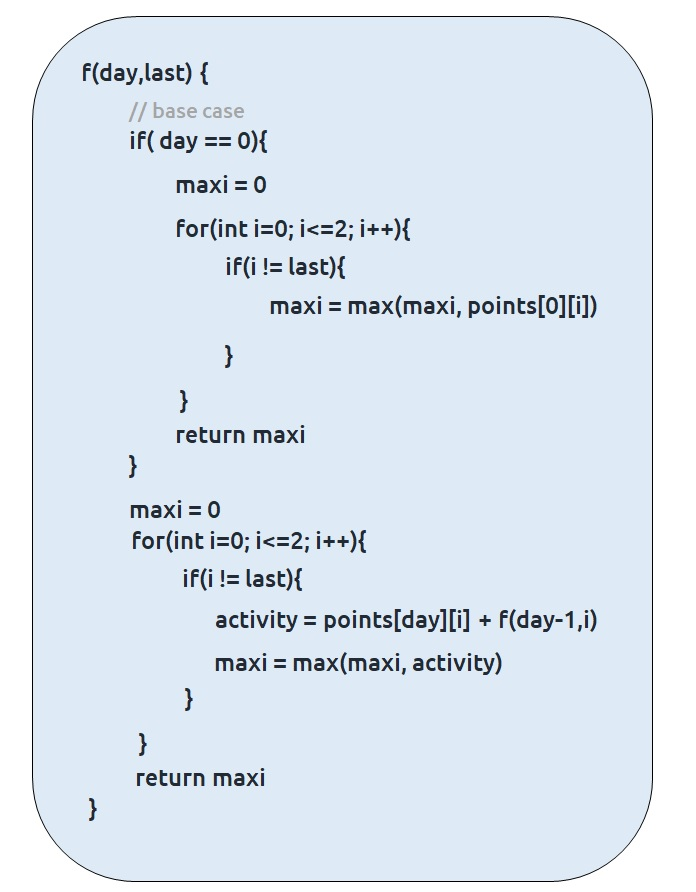Steps to memoize a recursive solution:

If we draw the recursion tree, we will see that there are overlapping subproblems. In order to convert a recursive solution to the following steps will be taken:

1. Create a dp array of size [n]. There are total ‘n’ days and for every day, there can be 4 choices (0,1,2 and 3). Therefore we take the dp array as dp[n].
2. Whenever we want to find the answer of particular parameters (say f(day,last)), we first check whether the answer is already calculated using the dp array(i.e dp[day][last]!= -1 ). If yes, simply return the value from the dp array.
3. If not, then we are finding the answer for the given value for the first time, we will use the recursive relation as usual but before returning from the function, we will set dp[day][last] to the solution we get.

Code:

## C++ Code

``````#include <bits/stdc++.h>

using namespace std;

int f(int day, int last, vector<vector<int>> &points,vector<vector<int>> &dp) {

if (dp[day][last] != -1) return dp[day][last];

if (day == 0) {
int maxi = 0;
for (int i = 0; i <= 2; i++) {
if (i != last)
maxi = max(maxi, points[i]);
}
return dp[day][last] = maxi;
}

int maxi = 0;
for (int i = 0; i <= 2; i++) {
if (i != last) {
int activity = points[day][i] + f(day - 1, i, points, dp);
maxi = max(maxi, activity);
}

}

return dp[day][last] = maxi;
}

int ninjaTraining(int n, vector < vector < int > > & points) {

vector < vector < int > > dp(n, vector < int > (4, -1));
return f(n - 1, 3, points, dp);
}

int main() {

vector < vector < int > > points = {{10,40,70},
{20,50,80},
{30,60,90}};

int n = points.size();
cout << ninjaTraining(n, points);
}``````

Output: 210

Time Complexity: O(N*4*3)

Reason: There are N*4 states and for every state, we are running a for loop iterating three times.

Space Complexity: O(N) + O(N*4)

Reason: We are using a recursion stack space(O(N)) and a 2D array (again O(N*4)). Therefore total space complexity will be O(N) + O(N) ≈ O(N)

## Java Code

``````import java.util.*;
class TUF {
static int f(int day, int last, int[][] points, int[][] dp) {

if (dp[day][last] != -1) return dp[day][last];

if (day == 0) {
int maxi = 0;
for (int i = 0; i <= 2; i++) {
if (i != last)
maxi = Math.max(maxi, points[i]);
}
return dp[day][last] = maxi;
}

int maxi = 0;
for (int i = 0; i <= 2; i++) {
if (i != last) {
int activity = points[day][i] + f(day - 1, i, points, dp);
maxi = Math.max(maxi, activity);
}

}

return dp[day][last] = maxi;
}

static int ninjaTraining(int n, int[][] points) {

int dp[][] = new int[n];
for (int[] row: dp)
Arrays.fill(row, -1);

return f(n - 1, 3, points, dp);
}

public static void main(String args[]) {

int[][] points = {{10,40,70},
{20,50,80},
{30,60,90}};

int n = points.length;
System.out.println(ninjaTraining(n, points));
}
}``````

Output: 210

Time Complexity: O(N*4*3)

Reason: There are N*4 states and for every state, we are running a for loop iterating three times.

Space Complexity: O(N) + O(N*4)

Reason: We are using a recursion stack space(O(N)) and a 2D array (again O(N*4)). Therefore total space complexity will be O(N) + O(N) ≈ O(N)

Steps to convert Recursive Solution to Tabulation one.

• Declare a dp[] array of size [n]
• First initialize the base condition values. We know that base condition arises when day = 0. Therefore, we can say that the following will be the base conditions
• dp = max(points, points)
• dp = max(points, points)
• dp = max(points, points)
• dp = max(points, points and points)
• Set an iterative loop which traverses dp array (from index 1 to n) and for every index set its value according to the recursive logic

Code:

## C++ Code

``````#include <bits/stdc++.h>

using namespace std;

int ninjaTraining(int n, vector < vector < int > > & points) {

vector < vector < int > > dp(n, vector < int > (4, 0));

dp = max(points, points);
dp = max(points, points);
dp = max(points, points);
dp = max(points, max(points, points));

for (int day = 1; day < n; day++) {
for (int last = 0; last < 4; last++) {
dp[day][last] = 0;
for (int task = 0; task <= 2; task++) {
if (task != last) {
int activity = points[day][task] + dp[day - 1][task];
dp[day][last] = max(dp[day][last], activity);
}
}
}

}

return dp[n - 1];
}

int main() {

vector<vector<int> > points = {{10,40,70},
{20,50,80},
{30,60,90}};
int n = points.size();
cout << ninjaTraining(n, points);
}``````

Output: 210

Time Complexity: O(N*4*3)

Reason: There are three nested loops

Space Complexity: O(N*4)

Reason: We are using an external array of size ‘N*4’’.

## Java Code

``````import java.util.*;
class TUF {
static int ninjaTraining(int n, int[][] points) {

int[][] dp = new int[n];
dp = Math.max(points, points);
dp = Math.max(points, points);
dp = Math.max(points, points);
dp = Math.max(points, Math.max(points, points));

for (int day = 1; day < n; day++) {
for (int last = 0; last < 4; last++) {
dp[day][last] = 0;
for (int task = 0; task <= 2; task++) {
if (task != last) {
int activity = points[day][task] + dp[day - 1][task];
dp[day][last] = Math.max(dp[day][last], activity);
}
}
}

}

return dp[n - 1];
}

public static void main(String args[]) {

int[][] points = {{10,40,70},
{20,50,80},
{30,60,90}};

int n = points.length;
System.out.println(ninjaTraining(n, points));
}
}``````

Output: 210

Time Complexity: O(N*4*3)

Reason: There are three nested loops

Space Complexity: O(N*4)

Reason: We are using an external array of size ‘N*4’’.

Part 3: Space Optimization

If we closely look the relation,

Here the task can be anything from 0 to 3 and day-1 is the previous stage of recursion. So in order to compute any dp array value, we only require the last row to calculate it.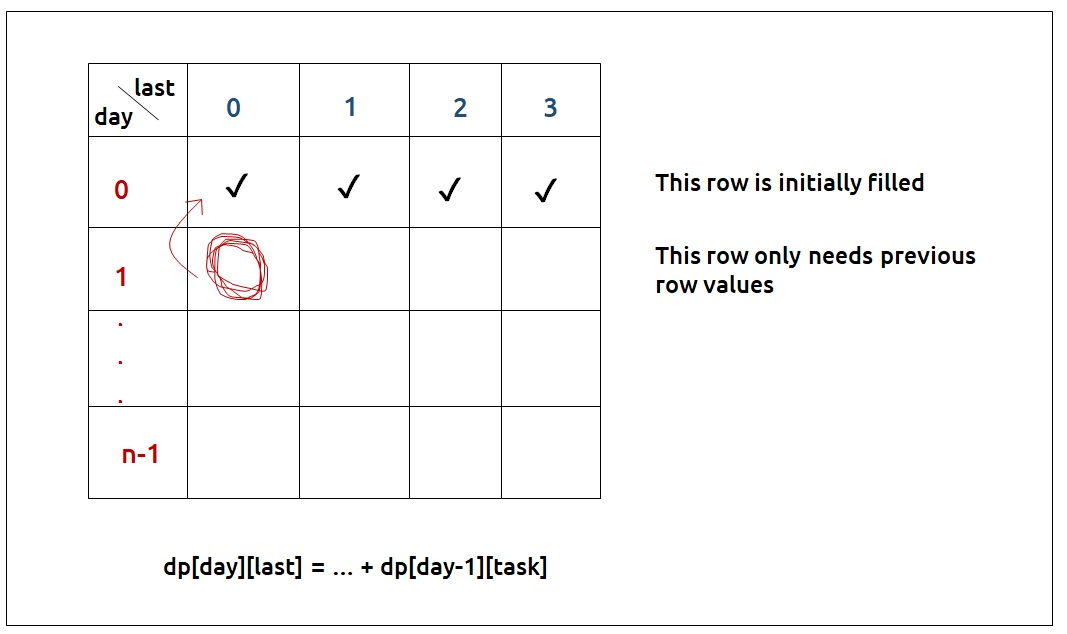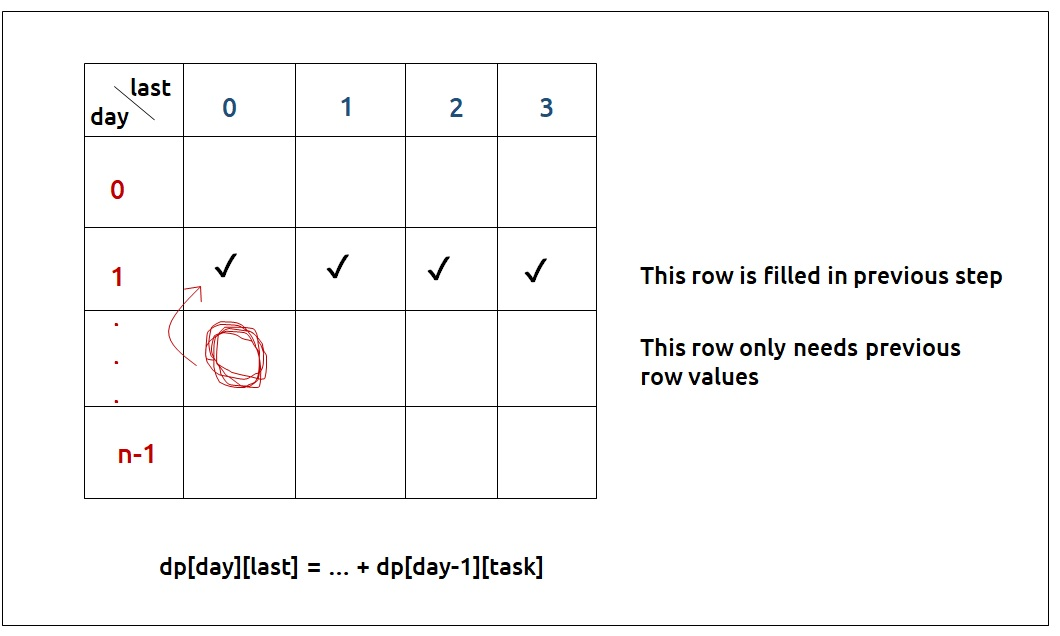• So rather than storing the entire 2D Array of size N*4, we can just store values of size 4(say prev).
• We can then take a dummy array, again of size 4 (say temp) and calculate the next row’s value using the array we stored in step 1.
• After that whenever we move to the next day, the temp array becomes our prev for the next step.
• At last prev will give us the answer.

Code:

## C++ Code

``````#include <bits/stdc++.h>

using namespace std;

int ninjaTraining(int n, vector < vector < int > > & points) {

vector < int > prev(4, 0);

prev = max(points, points);
prev = max(points, points);
prev = max(points, points);
prev = max(points, max(points, points));

for (int day = 1; day < n; day++) {

vector < int > temp(4, 0);
for (int last = 0; last < 4; last++) {
temp[last] = 0;
for (int task = 0; task <= 2; task++) {
if (task != last) {
}
}
}

prev = temp;

}

return prev;
}

int main() {

vector<vector<int> > points = {{10,40,70},
{20,50,80},
{30,60,90}};

int n = points.size();
cout << ninjaTraining(n, points);
}``````

Output:

210

Time Complexity: O(N*4*3)

Reason: There are three nested loops

Space Complexity: O(4)

Reason: We are using an external array of size ‘4’ to store only one row.

## Java Code

``````import java.util.*;
class TUF {
static int ninjaTraining(int n, int[][] points) {

int prev[] = new int;

prev = Math.max(points, points);
prev = Math.max(points, points);
prev = Math.max(points, points);
prev = Math.max(points, Math.max(points, points));

for (int day = 1; day < n; day++) {

int temp[] = new int;
for (int last = 0; last < 4; last++) {
temp[last] = 0;
for (int task = 0; task <= 2; task++) {
if (task != last) {
}
}
}

prev = temp;

}

return prev;
}

public static void main(String args[]) {

int[][] points = {{10,40,70},
{20,50,80},
{30,60,90}};

int n = points.length;
System.out.println(ninjaTraining(n, points));
}
}``````

Output:

210

Time Complexity: O(N*4*3)

Reason: There are three nested loops

Space Complexity: O(4)

Reason: We are using an external array of size ‘4’ to store only one row.

Special thanks to Anshuman Sharma for contributing to this article on takeUforward. If you also wish to share your knowledge with the takeUforward fam, please check out this article